The current in the arm CD of the circuit will be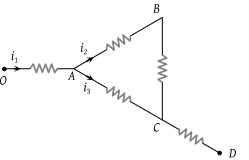(1) ${i}_{1}+{i}_{2}$

(2) ${i}_{2}+{i}_{3}$

(3) ${i}_{1}+{i}_{3}$

(4) ${i}_{1}-{i}_{2}+{i}_{3}$

Concept Questions :-

Kirchoff's current law
High Yielding Test Series + Question Bank - NEET 2020

Difficulty Level:

Two non-ideal identical batteries are connected in parallel. Consider the following statements :

(i) The equivalent e.m.f. is smaller than either of the two e.m.f.s

(ii) The equivalent internal resistance is smaller than either of the two internal resistances

(1) Both (i) and (ii) are correct

(2) (i) is correct but (ii) is wrong

(3) (ii) is correct but (i) is wrong

(4) Both (i) and (ii) are wrong

Concept Questions :-

Grouping of cells
High Yielding Test Series + Question Bank - NEET 2020

Difficulty Level:

Consider the circuit shown in the figure. The current I3 is equal to :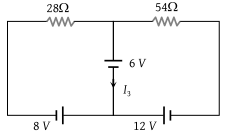(1) 5 amp

(2) 3 amp

(3) –3 amp

(4) –5/6 amp

Concept Questions :-

Kirchoff's voltage law
High Yielding Test Series + Question Bank - NEET 2020

Difficulty Level:

If ${V}_{AB}=4V$ in the given figure, then resistance X will be :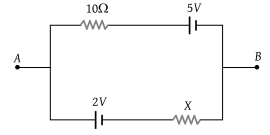(1) 5 Ω

(2) 10 Ω

(3) 15 Ω

(4) 20 Ω

Concept Questions :-

Kirchoff's voltage law
High Yielding Test Series + Question Bank - NEET 2020

Difficulty Level:

The number of dry cells, each of e.m.f. 1.5 volt and internal resistance 0.5 ohm that must be joined in series with a resistance of 20 ohm so as to send a current of 0.6 ampere through the circuit is

(1) 2

(2) 8

(3) 10

(4) 12

Concept Questions :-

Grouping of cells
High Yielding Test Series + Question Bank - NEET 2020

Difficulty Level:

For driving a current of 2 A for 6 minutes in a circuit, 1000 J of work is to be done. The e.m.f. of the source in the circuit is

(1) 1.38 V

(2) 1.68 V

(3) 2.04 V

(4) 3.10 V

Concept Questions :-

Heating effect of current
High Yielding Test Series + Question Bank - NEET 2020

Difficulty Level:

Two batteries of e.m.f. 4V and 8 V with internal resistances 1 Ω and 2 Ω are connected in a circuit with a resistance of 9 Ω as shown in figure. The current and potential difference between the points P and Q are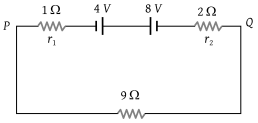(1)

(2)

(3)

(4) $\frac{1}{2}A\text{\hspace{0.17em}\hspace{0.17em}and\hspace{0.17em}\hspace{0.17em}}12V$

Concept Questions :-

Grouping of cells
High Yielding Test Series + Question Bank - NEET 2020

Difficulty Level:

Four identical cells each having an electromotive force (e.m.f.) of 12V, are connected in parallel. The resultant electromotive force (e.m.f.) of the combination is :

(1) 48 V

(2) 12 V

(3) 4 V

(4) 3 V

Concept Questions :-

Grouping of cells
High Yielding Test Series + Question Bank - NEET 2020

Difficulty Level:

The internal resistance of a cell of e.m.f. 12V is $5×{10}^{-2}\text{\hspace{0.17em}}\Omega$. It is connected across an unknown resistance. The voltage across the cell, when a current of 60 A is drawn from it, is :

(1) 15 V

(2) 12 V

(3) 9 V

(4) 6 V

Concept Questions :-

Emf and terminal voltage
High Yielding Test Series + Question Bank - NEET 2020

Difficulty Level:

The current in the given circuit is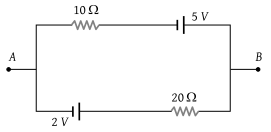(1) 0.1 A

(2) 0.2 A

(3) 0.3 A

(4) 0.4 A

Concept Questions :-

Kirchoff's voltage law